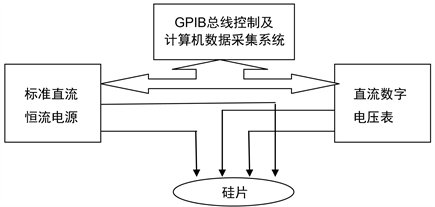# 直排四探针法测量硅片电阻率及不确定度评定Measuring Resistivity of Silicon Wafers with Four-Point Probe Method and Evaluation of Uncertainty in Measurement

• 全文下载: PDF(463KB)    PP.1041-1047   DOI: 10.12677/MS.2019.912128
• 下载量: 128  浏览量: 164

Resistivity measuring instruments with four-point probe is widely used in semiconductor industry, and it is a common device for measuring resistivity of semiconductor materials. In this paper, the measurement conditions and applicable calculation formulas for different samples are briefly de-scribed, and the uncertainty evaluation of the precise measurement results of wafer is discussed in detail.

1. 经典直排四探针测试仪的基本原理

$\rho =2\pi S\frac{U}{I}$ (1)Figure 1. Schematic diagram of classical four-point probe instrument

2. 四探针测试仪的实际应用

${\rho }_{23}={\rho }_{T}×{F}_{T}$ (2)

${\rho }_{T}$ 是实际温度T下测得的电阻率，Ω∙cm。

${F}_{T}$ 是温度修正因子。

${F}_{T}=1-{C}_{T}\left(T-23\right)$ (3)

T是被测样品的实际温度，℃。

${F}_{SP}=1+1.082\left(1-{\stackrel{¯}{S}}_{2}/\stackrel{¯}{S}\right)$ (4)

$\stackrel{¯}{S}=1/3\left({\stackrel{¯}{S}}_{1}+{\stackrel{¯}{S}}_{2}+{\stackrel{¯}{S}}_{3}\right)$

$\stackrel{¯}{S}$ 是探针间距的平均值。

${\stackrel{¯}{S}}_{1}$${\stackrel{¯}{S}}_{2}$${\stackrel{¯}{S}}_{3}$ 分别是探针的三个间距的十次测量平均值。

${\rho }_{23}=\frac{\stackrel{¯}{V}}{I}×W×F\left(W/\stackrel{¯}{S}\right)×F\left(\stackrel{¯}{S}/D\right)×{F}_{SP}×{F}_{T}$ (5)

$F\left(W/\stackrel{¯}{S}\right)$ 是厚度修正系数。

$F\left(\stackrel{¯}{S}/D\right)$ 是直径修正系数。

${F}_{SP}$ 是探针修正系数。

${F}_{T}$ 是温度修正系数。

3. 电阻率测量结果的不确定度评定  

1) 测量重复性引入的不确定度分量 ${u}_{A}$Table 1. Measured resistivity values data

${u}_{A}=u\left(\stackrel{¯}{\rho }\right)=\frac{u\left(\rho \right)}{\sqrt{10}}=\frac{1}{\sqrt{\left(10-1\right)×10}}×\sqrt{{\sum }_{k=1}^{10}{\left({\rho }_{k}-\stackrel{¯}{\rho }\right)}^{2}}=0.000005\text{\hspace{0.17em}}\Omega \cdot \text{cm}$

${u}_{A}=\frac{0.000005}{0.009462}×100=0.053%$

2) B类不确定度分量 ${u}_{B}$

a) 电子设备引入的分量 ${u}_{B1},{u}_{B2}$Table 2. Nano voltmeter calibration data

${u}_{B1}=\frac{0.00007}{2×5.00004}×100=0.0007%$Table 3. Constant current source calibration data

${u}_{B2}=\frac{0.02}{2}=0.01%$

b) 温度变化带来的不确定度分量 ${u}_{B3}$

${u}_{B3}=0.0035%$

c) 测厚仪传递的不确定度分量 ${u}_{B4}$

$T=\stackrel{¯}{x}+\Delta {t}_{S}$

$\stackrel{¯}{x}$ 为测厚仪的读数，即测量结果。 $\Delta {t}_{S}$ 为测厚仪的误差对测量结果的影响，其数学期望值为零，但需考虑其不确定度。

629.1 μm 629.0 μm 628.9 μm

${u}_{\text{1}}\left(t\right)=u\left(\stackrel{¯}{x}\right)=s\left(\stackrel{¯}{x}\right)=0.04\text{\hspace{0.17em}}\mu \text{m}$

${u}_{2}\left(t\right)=\frac{0.6}{\sqrt{3}}=0.346\text{\hspace{0.17em}}\mu \text{m}$

d) 探针头引入的不确定度分量 ${u}_{B5}$

$\begin{array}{c}{u}_{C}=\sqrt{{u}_{A}^{2}+{u}_{B1}^{2}+{u}_{B2}^{2}+{u}_{B3}^{2}+{u}_{B4}^{2}+{u}_{B5}^{2}}\\ =\sqrt{{0.053}^{2}+{0.0007}^{2}+{0.01}^{2}+{0.0035}^{2}+{0.055}^{2}+{0.076}^{2}}\\ =0.11%\end{array}$

$U=k{u}_{C}=0.22%$

$\begin{array}{c}{u}_{B}=\sqrt{{u}_{B1}^{2}+{u}_{B2}^{2}+{u}_{B3}^{2}+{u}_{B4}^{2}+{u}_{B5}^{2}}\\ =\sqrt{{0.00065}^{2}+{0.01}^{2}+{0.166}^{2}+{0.055}^{2}+{0.076}^{2}}\\ =0.19%\end{array}$

$\begin{array}{c}{u}_{C\mathrm{max}}=\sqrt{{u}_{A}^{2}+{u}_{B1}^{2}+{u}_{B2}^{2}+{u}_{B3}^{2}+{u}_{B4}^{2}+{u}_{B5}^{2}}\\ =\sqrt{{0.2}^{2}+{0.00065}^{2}+{0.01}^{2}+{0.166}^{2}+{0.055}^{2}+{0.076}^{2}}\\ =0.28%\end{array}$

4. 结论

  全国半导体设备和材料标准化技术委员会, 材料分会. 半导体材料标准汇编(2014版) [M]. 北京: 中国标准出版社, 2014.  半导体设备和材料国际组织中国标准化协会SEMI标准年鉴[M]. 北京: 中国标准出版社, 1987.  刘玉岭, 等. 微电子技术工程[M]. 北京: 电子工业出版社, 2004.  陈奕钦. 测量不确定度93国际指南应用实例[M]. 北京: 中国计量出版社, 1998.  测量不确定度评定与表示指南[M]. 北京: 中国计量出版社, 1997.